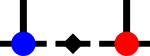SyTenstensor_matrixop.h File Reference

Matrix operations for smart tensors. More...

#include "inc/stensor/stensor_base.h"Include dependency graph for stensor_matrixop.h:This graph shows which files directly or indirectly include this file:

## Namespaces

syten::STensorImpl
Contains all ‘smart’ tensor related classes and functions.

## Functions

Vec< SDef > syten::STensorImpl::diagonal (STensor const &in)
Returns the diagonal entries of the rank-2 input tensor. More...

void syten::STensorImpl::diagonal_signs_to_largest (STensor &in)
Finds the diagonal element with the largest amplitude and sets every element whose real part does not have the same sign as this element to zero. More...

STensor syten::STensorImpl::exp (STensor in, SDef scalar=1.)
Takes the exponential of a hermitian rank-2 tensor multiplied by a scalar prefactor by direct exponentiation after mapping it to matrix form (outgoing leg first, incoming leg second in fermionic order). More...

STensor syten::STensorImpl::hadamard (STensor const &a, STensor b)
Takes the Hadamard product of two rank-2 tensors. More...

STensor syten::STensorImpl::hadamard_inv_diffdiff (STensor const &s, STensor x, SBasisId const &j_basis, SBasisId const &i_basis, bool minus)
Given a diagonal square tensor s and a square tensor x, approximates $$x_i^j \left( \frac{1}{s_j - s_i} \pm \frac{1}{s_j + s_i} \right)$$. More...

STensor syten::STensorImpl::herm_to_symmetrise (STensor in)
Takes the hermitian conjugate and adapts the bases such that addition with the input tensor results in a symmetric tensor. More...

STensor syten::STensorImpl::hermitize_by_copy (STensor in, SBasisId row_id, SBasisId col_id)
Hermitizes the input tensor by copying the elements from the upper triangular part complex-conjugated to the lower triangular part. More...

STensor syten::STensorImpl::invert_diagonal (STensor in)
Inverts the diagonal entries of the rank-2 input tensor. More...

STensor syten::STensorImpl::log (STensor in)
Given a real rank-0 STensor, returns its natural logarithm. More...

STensor syten::STensorImpl::log2 (STensor in)
Given a real rank-0 STensor, returns its base-2 logarithm. More...

std::tuple< SRDef, STensor > syten::STensorImpl::pseudo_invert (STensor const &in)
Inverts the tensor using a SVD and returns the condition number of the result, which is obtained as the ratio of the largest and smallest inverted singular value. More...

STensor syten::STensorImpl::sqrt (STensor in)
Takes the square root of a diagonal rank-2 or rank-0 tensor after mapping it to matrix form (outgoing leg first, incoming leg second in fermionic order). More...

STensor syten::STensorImpl::trace (STensor const &in)
Calculates the trace of a tensor. More...

## Detailed Description

Matrix operations for smart tensors.